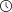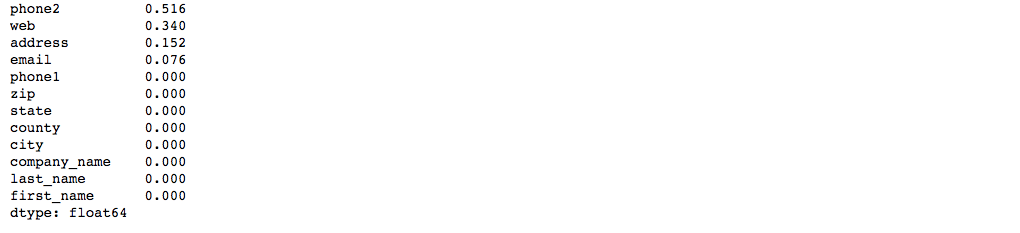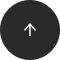- 8 minutes read

# 12 Python Tips and Tricks That Every Data Scientist Should Know

You already have some foundational knowledge of Python for data science. But do you write your code efficiently? Check out these tips and tricks to supercharge your Python skills.

### How to Write Efficient Python Code

In this article, we'll take a look at some tricks that will help you write fast and efficient Python code. I'll start with how to optimize code that involves the `pandas` library. If you want to refresh your knowledge of pandas, check out our Introduction to Python for Data Science course.

Afterwards, I'll move on to some other general Python best practices, including list comprehensions, enumerators, strings concatenation, and more.

### 1. Determining the Percentage of Missing Data

For illustration, I'm going to use a synthetic dataset with the contact information of 500 fictitious subjects from the US. Let's imagine that this is our client base. Here's what the dataset looks like:

```clients.head()
```As you can see, it includes information on each person's first name, last name, company name, address, city, county, state, zip code, phone numbers, email, and web address.

Our first task is to check for missing data. You can use `clients.info()` to get an overview of the number of complete entries in each of the columns. However, if you want a clearer picture, here's how you can get the percentage of missing entries for each of the features in descending order:

```# Getting percentange of missing data for each column
(clients.isnull().sum()/clients.isnull().count()).sort_values(ascending=False)
```As you may recall, `isnull()` returns an array of True and False values that indicate whether a given entry is present or missing, respectively. In addition, True is considered as 1 and False is considered as 0 when we pass this boolean object to mathematical operations. Thus, `clients.isnull().sum()` gives us the number of missing values in each of the columns (the number of True values), while `clients.isnull().count()` is the total number of values in each column.

After we divide the first value by the second and sort our results in descending order, we get the percentage of missing data entries for each column, starting with the column that has the most missing values. In our example, we see that we miss the second phone number for 51.6% of our clients.

### 2. Finding a Unique Set of Values

There's a standard way to get a list of unique values for a particular column: `clients['state'].unique()`. However, if you have a huge dataset with millions of entries, you might prefer a much faster option:

```# Getting percentange of missing data for each column
(clients.isnull().sum()/clients.isnull().count()).sort_values(ascending=False)
```This way, you drop all the duplicates and keep only the first occurrence of each value. We've also sorted the results to check that each state is indeed mentioned only once.

### 3. Joining Columns

Often, you might need to join several columns with a specific separator. Here's an easy way to do this:

```# Joining columns with first and last name
clients['name'] = clients['first_name'] + ' ' + clients['last_name']
```
```clients['name'].head()
```As you can see, we combined the `first_name` and `last_name` columns into the name column, where the first and last names are separated by a space.

### 4. Splitting Columns

And what if we need to split columns instead? Here's an efficient way to split one column into two columns using the first space character in a data entry:

```# Getting first name from the 'name' column
clients['f_name'] = clients['name'].str.split(' ', expand = True)
```
```# Getting last name from the 'name' column
clients['l_name'] = clients['name'].str.split(' ', expand = True)
```

Now we save the first part of the name as the `f_name` column and the second part of the name as a separate `l_name` column.

### 5. Checking if Two Columns Are Identical

Since we've practiced joining and splitting columns, you might have noticed that we now have two columns with the first name (`first_name` and `f_name`) and two columns with the last name (`last_name` and `l_name`). Let's quickly check if these columns are identical.

First, note that you can use `equals()` to check the equality of columns or even entire datasets:

```# Checking if two columns are identical with .equals()
clients['first_name'].equals(clients['f_name'])
```
```True
```

You'll get a `True` or `False` answer. But what if you get `False` and want to know how many entries don't match? Here's a simple way to get this information:

```# Checking how many entries in the initial column match the entries in the new column
(clients['first_name'] == clients['f_name']).sum()
```
```500
```

We've started with getting the number of entries that do match. Here, we again utilize the fact that True is considered as 1 in our calculations. We see that 500 entries from the `first_name` column match the entries in the `f_name` column. You may recall that 500 is the total number of rows in our dataset, so this means all entries match. However, you may not always remember (or know) the total number of entries in your dataset. So, for our second example, we get the number of entries that do not match by subtracting the number of matching entries from the total number of entries:

```# Checking how many entries in the initial column DO NOT match the entries in the new column
clients['last_name'].count() - (clients['last_name'] == clients['l_name']).sum()
```
```0
```

### 6. Grouping Data

To demonstrate how we can group data efficiently in pandas, let's first create a new column with the providers of email services. Here, we can use the trick for splitting columns that you're already familiar with:

```# Creating new columb with the email service providers
clients['email_provider'] = clients['email'].str.split('@', expand = True)
```
```clients['email_provider'].head()
```Now let's group the clients by state and `email_provider`:

```# Grouping clients by state and email provider
clients.groupby('state')['email_provider'].value_counts()
```We've now got a data frame that uses several levels of indexing to provide access to each observation (known as multi-indexing).

### 7. Unstack

Sometimes, you'll prefer to transform one level of the index (like `email_provider`) into the columns of your data frame. That's exactly what `unstack()` does. It's better to explain this with an example. So, let's unstack our code above:

```# Moving 'Mail providers' to the column names
clients.groupby('state')['email_provider'].value_counts().unstack().fillna(0)
```As you can see, the values for the email service providers are now the columns of our data frame.

Now it's time to move on to some other general Python tricks beyond `pandas`.

### 8. Using List Comprehensions

List comprehension is one of the key Python features, and you may already be familiar with this concept. Even if you are, here's a quick reminder of how list comprehensions help us create lists much more efficiently.:

```# Inefficient way to create new list based on some old list
squares = []
for x in range(5):
squares.append(x**2)
print(squares)
```
```[0, 1, 4, 9, 16]
```
```# Efficient way to create new list based on some old list
squares = [x**2 for x in range(5)]
print(squares)
```
```[0, 1, 4, 9, 16]
```

### 9. Concatenating Strings

When you need to concatenate a list of strings, you can do this using a for loop and adding each element one by one. However, this would be very inefficient, especially if the list is long. In Python, strings are immutable, and thus the left and right strings would have to be copied into the new string for every pair of concatenation.

A better approach is to use the `join()` function as shown below:

```# Naive way to concatenate strings
sep = ['a', 'b', 'c', 'd', 'e']
joined = ""
for x in sep:
joined += x
print(joined)
```
```abcde
```
```# Joining strings
sep = ['a', 'b', 'c', 'd', 'e']
joined = "".join(sep)
print(joined)
```
```abcde
```

### 10. Using Enumerators

How would you print a numbered list of the world's richest people? Maybe you'd consider something like this:

```# Inefficient way to get numbered list
the_richest = ['Jeff Bezos', 'Bill Gates', 'Warren Buffett', 'Bernard Arnault & family', 'Mark Zuckerberg']
i = 0
for person in the_richest:
print(i, person)
i+=1
```However, you can do the same with less code using the `enumerate()` function:

```# Efficient way to get numbered list
the_richest = ['Jeff Bezos', 'Bill Gates', 'Warren Buffett', 'Bernard Arnault & family', 'Mark Zuckerberg']
for i, person in enumerate(the_richest):
print(i, person)
```Enumerators can be very useful when you need to iterate through a list while keeping track of the list items' indices.

### 11. Using ZIP When Working with Lists

Now, how would you proceed if you needed to combine several lists with the same length and print out the result? Again, here is a more generic and "Pythonic" way to get the desired result by utilizing `zip()`:

```# Inefficient way to combine two lists
the_richest = ['Jeff Bezos', 'Bill Gates', 'Warren Buffett', 'Bernard Arnault & family', 'Mark Zuckerberg']
fortune = ['\$112 billion', '\$90 billion', '\$84 billion', '\$72 billion', '\$71 billion']
for i in range(len(the_richest)):
person = the_richest[i]
amount = fortune[i]
print(person, amount)
``````# Efficient way to combine two lists
the_richest = ['Jeff Bezos', 'Bill Gates', 'Warren Buffett', 'Bernard Arnault & family', 'Mark Zuckerberg']
fortune = ['\$112 billion', '\$90 billion', '\$84 billion', '\$72 billion', '\$71 billion']
for person, amount in zip(the_richest,fortune):
print(person, amount)
```Possible applications of the `zip()` function include all the scenarios that require mapping of groups (e.g., employees and their wage and department info, students and their marks, etc).

If you need to recap working with lists and dictionaries, you can do that here online.

### 12. Swapping Variables

When you need to swap two variables, the most common way is to use a third, temporary variable. However, Python allows you to swap variables in just one line of code using tuples and packing/unpacking:

```# Swapping variables)
a = "January"
b = "2019"
print(a, b)
a, b = b, a
print(b, a)
```
```January 2019
January 2019
```

### Wrap-Up

Awesome! Now you're familiar with some useful Python tips and tricks that data scientists use in their day-to-day work. These tips should help you make your code more efficient and even impress your potential employers.

However, aside from using different tricks, it's also crucial for a data scientist to have a solid foundation in Python. Be sure to check out our Introduction to Python for Data Science course if you need a refresher; it covers the basics of pandas and `matplotlib`—the key Python libraries for data science—as well as other basic concepts you need for working with data in Python.